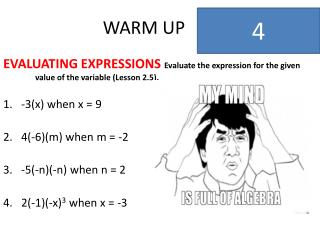DownloadDownload PresentationWARM UP

# WARM UP

Download Presentation## WARM UP

- - - - - - - - - - - - - - - - - - - - - - - - - - - E N D - - - - - - - - - - - - - - - - - - - - - - - - - - -
##### Presentation Transcript

1. WARM UP 4 EVALUATING EXPRESSIONS Evaluate the expression for the given value of the variable (Lesson 2.5). -3(x) when x = 9 4(-6)(m) when m = -2 -5(-n)(-n) when n = 2 2(-1)(-x)3 when x = -3

2. WARM UP 3 EVALUATING EXPRESSIONS Evaluate the expression for the given value of the variable (Lesson 2.5). -3(x) when x = 9 4(-6)(m) when m = -2 -5(-n)(-n) when n = 2 2(-1)(-x)3 when x = -3

3. WARM UP 2 EVALUATING EXPRESSIONS Evaluate the expression for the given value of the variable (Lesson 2.5). -3(x) when x = 9 4(-6)(m) when m = -2 -5(-n)(-n) when n = 2 2(-1)(-x)3 when x = -3

4. WARM UP 1 EVALUATING EXPRESSIONS Evaluate the expression for the given value of the variable (Lesson 2.5). -3(x) when x = 9 4(-6)(m) when m = -2 -5(-n)(-n) when n = 2 2(-1)(-x)3 when x = -3

5. WARM UP 0 EVALUATING EXPRESSIONS Evaluate the expression for the given value of the variable (Lesson 2.5). -3(x) when x = 9 4(-6)(m) when m = -2 -5(-n)(-n) when n = 2 2(-1)(-x)3 when x = -3